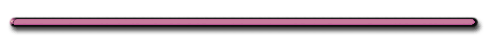Intro to Finding Resultants

Pre - Class Questions 1 - 3

Review – Finding Resultants (Fnet)
0, 180., 90. degrees

Resultant - Single force that produces the same result as 2 or more forces

1. Angle between vectors below = 0 degrees

(Same Direction)

Fnet = 9.0 N East        Fnet = 12 N Right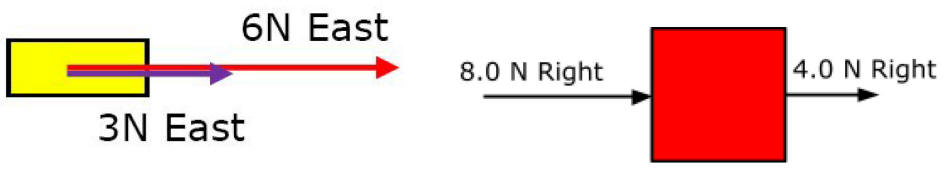ADD Forces when angle = 0 deg

2. Angle between vectors below = 180 deg.

Fnet = 40 N East (Subtract Forces)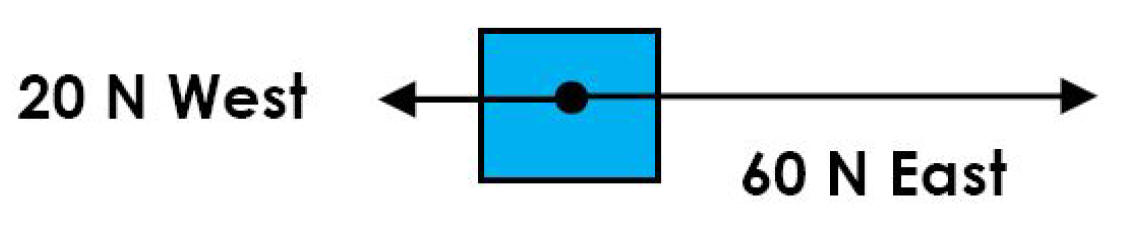3. Naming Direction with Angles

The vector on the upper right is 50 degrees N of E.
Name the rest of the directions.

 35 degrees N of W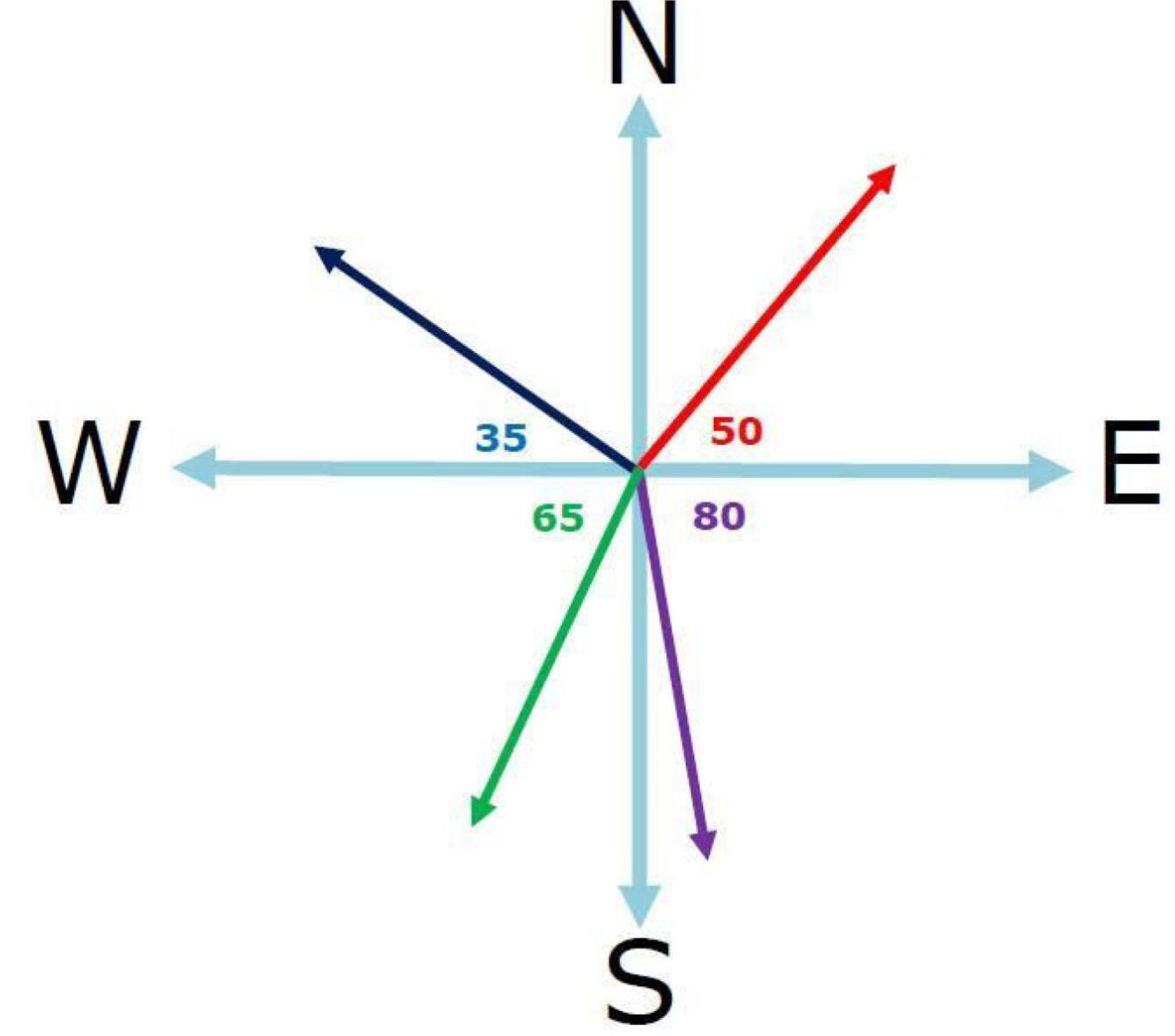65 deg. S of W 80 deg. S of E

4. Angle between vectors = 90 degrees. Use TRIG to find the direction.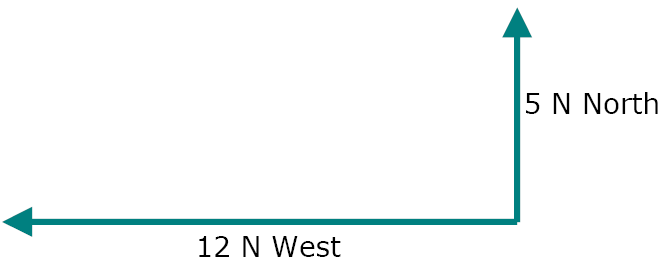2. Make a parallelogram3. Draw diagonal (resultant)4. Find magnitude & direction of vector

1. Use a2 + b2 = c2 (To find magnitude)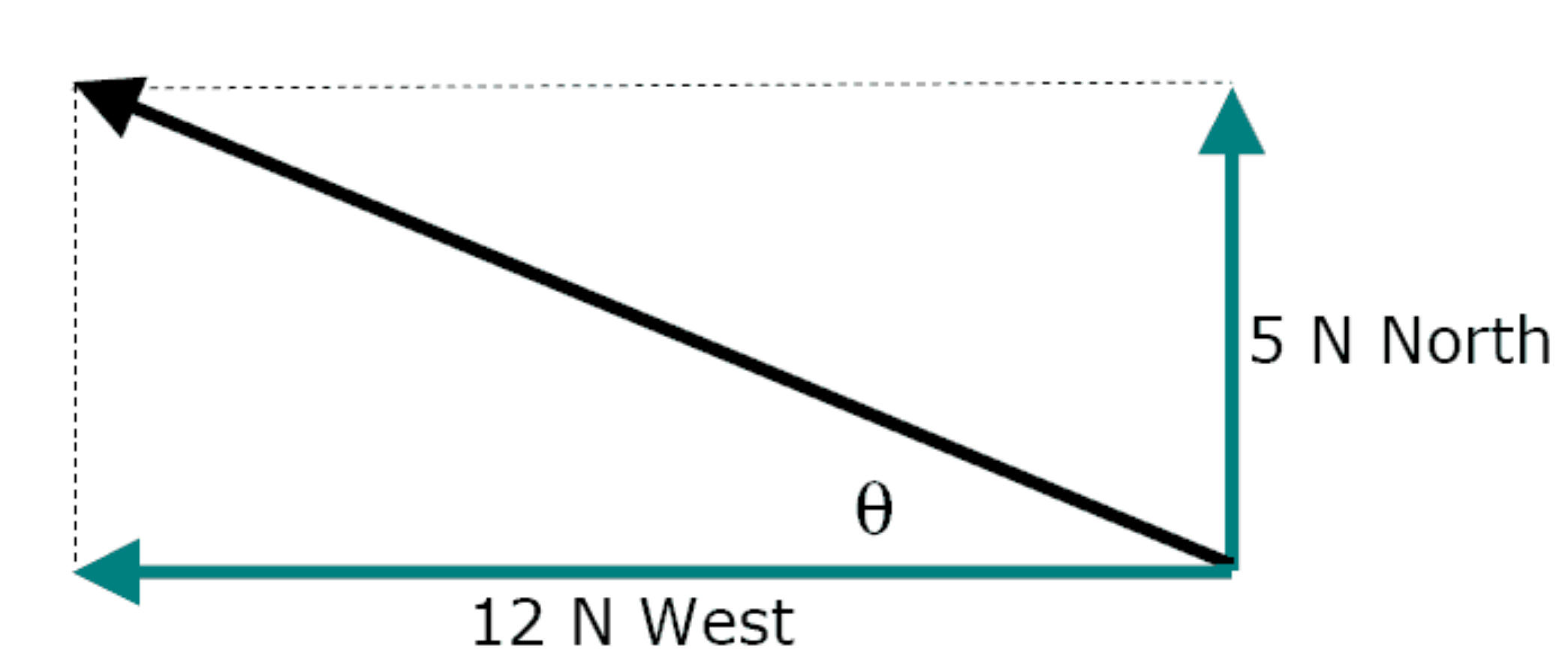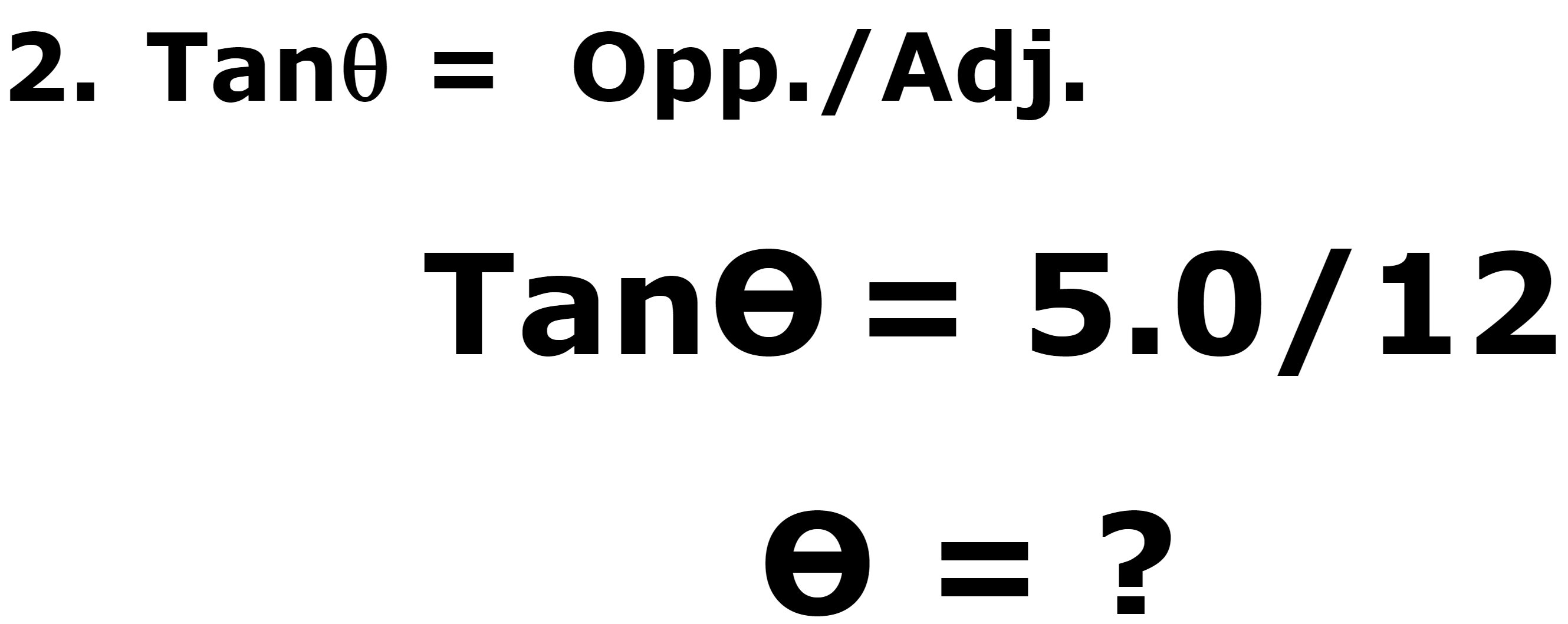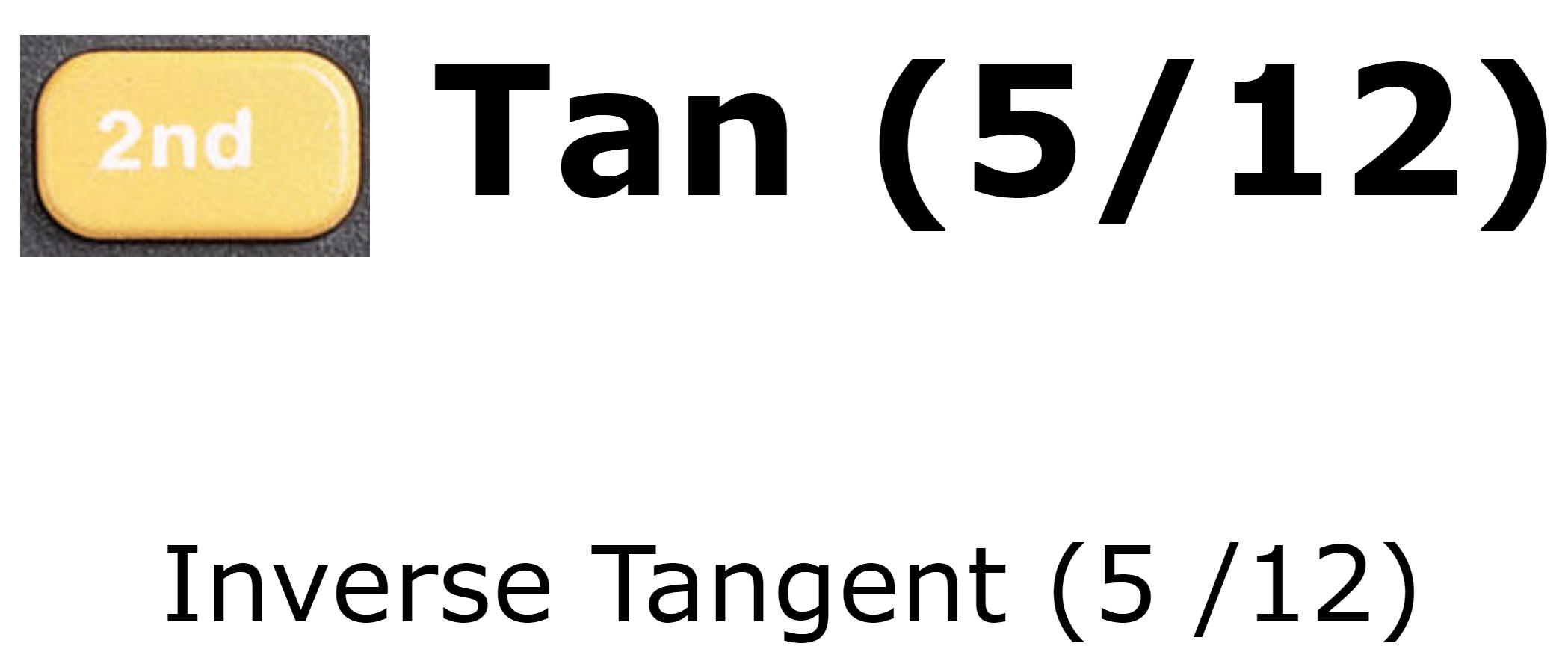5. 10. nt east and 10. nt south act on an object.

Find FnetFinding Fnet Using Components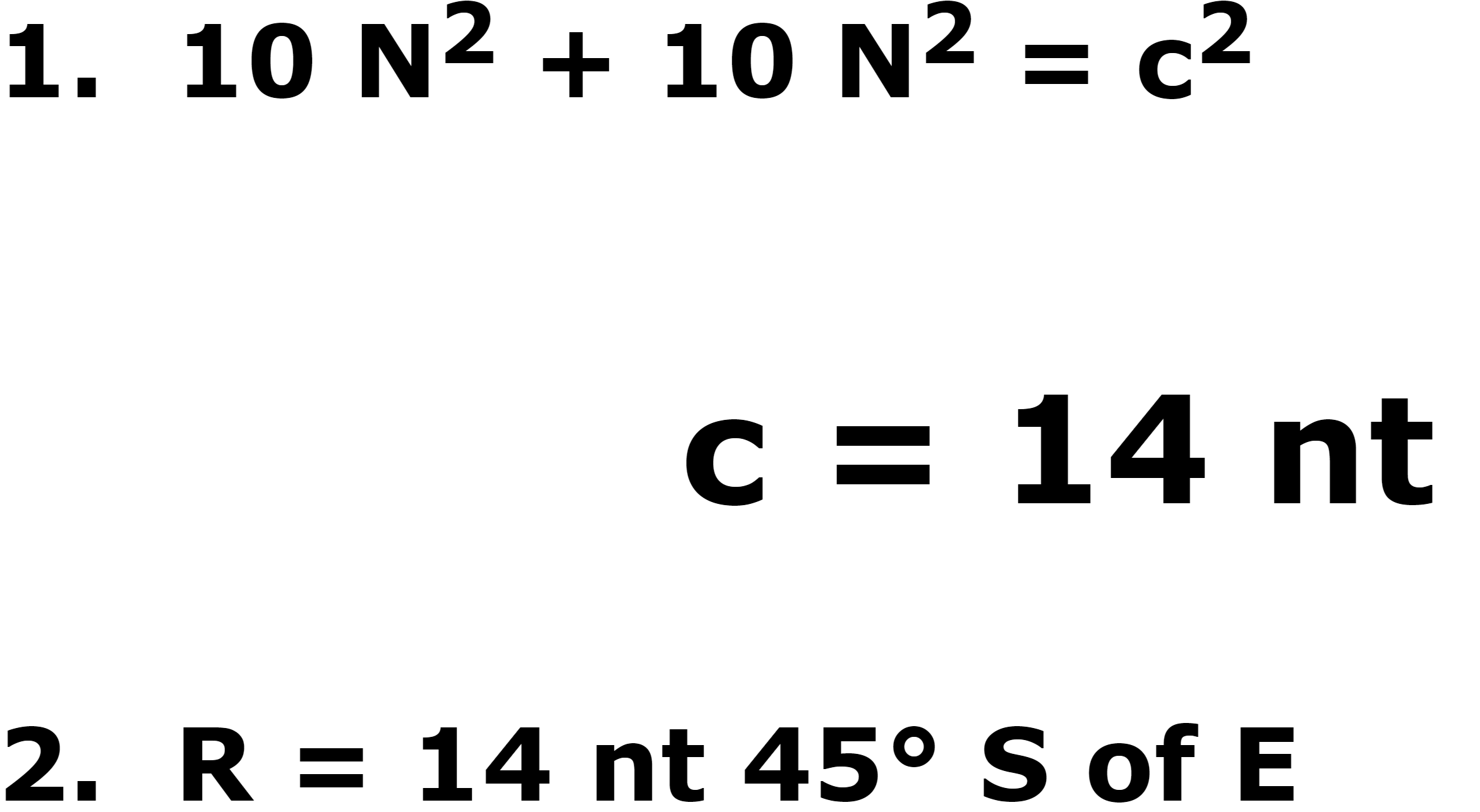Video Instruction

showmethephysics.com

Find the resultants
of these 2 vectors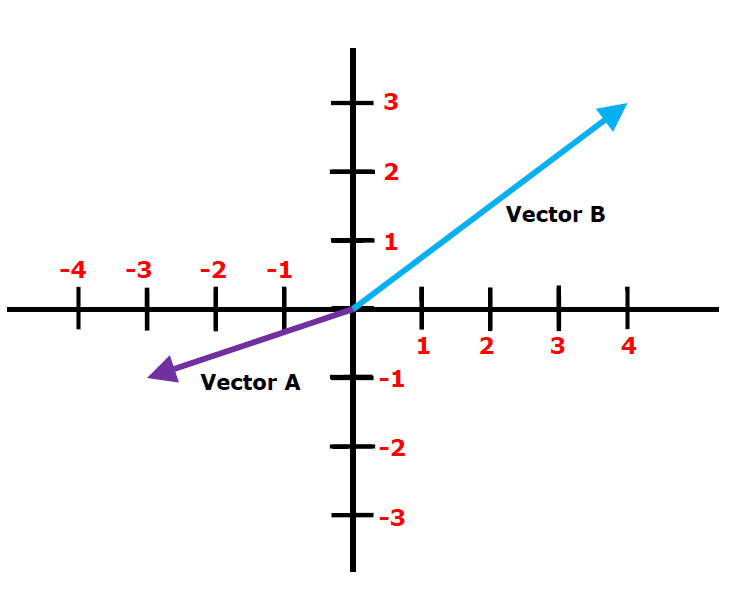When theta is NOT 0, 90 or 180 it is more useful to find Fnet (Resultant) by adding components

Step 1. Find Components of all vectors (Use neg. and pos.)

Find Fy and Fx of resultant

Fx = 4.0 N - 3.0 N= 1.0N

Fy = 3.0 N - 1.0 N= 2.0NFx = 1.0 N    Fy = 2.0 NX Y Vector B 4.0 N 3.0 N Vector A - 3.0 N -1.0 N Fnet 1.0 N 2.0 N

3. Use the pythagorean theorum to get the magnitude.

R = (1.0 N)2 + (2.0 N)2 = 2.2 N

4. Use tangent to get the direction

Tangent(theta) = Fy/Fx = 2.0 N/1.0 N

theta = 63 degrees

R = 2.2 N 63 degrees N of E

Ex) 5.0 N North & 3.0 N 30° S of E act concurrently (on a single point).

Find the Fx and Fy
of resultant in terms of theta

1. Sketch VectorsFind Fnet by adding components and using Tangent. Make a Table!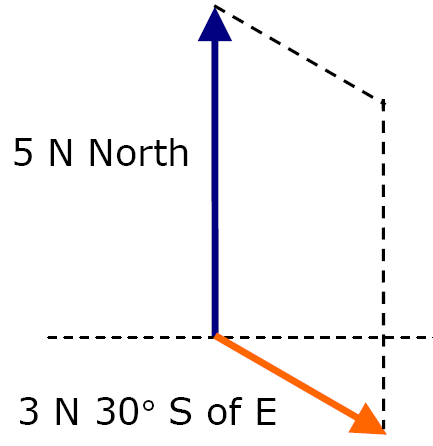1. Combine Fx and Fy

ΣFx = 0 + 3Ncos30

ΣFx = 2.6 N

ΣFy = 5.0N - 3.0Nsin30

ΣFy = 3.5 N

 X Y Vector 1 0 +5 N Vector 2 2.6 N -1.5 N Fnet 2.6 N 3.5 N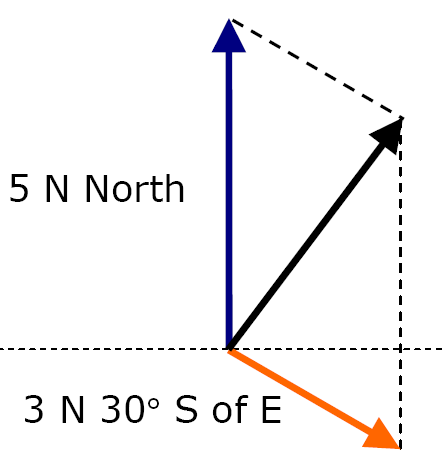Pyth. Theorem and Tangent

(2.6 N)2 + (3.5 N)2 = R2

tan(theta) = Fy/Fx = (3.5 N/2.6 N)

R = 4.5 N 53° N of E

E = 4.5 N 53° S of W

Quick Resultants Review

0 deg. - Same direction - ADD

180 deg. - Opp. Direction - Subtract

90 degrees - Pyth.

 RankingTask Rank the resultants from SMALLEST to LARGEST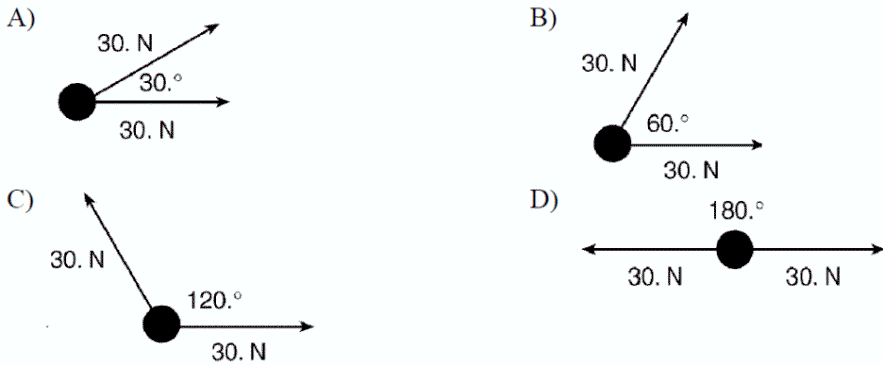showmehysics.com

AP Question

Hewitt Question

Ex) Relative to the ground, an airplane gains speed when it encounters wind from behind, and loses speed when it encounters wind head on.

When it encounters wind at a right angle to the direction it is pointing, its speed relative to the ground below

A. increases.

B. decreases.

C. is the same as if there were no wind.

Increases1st Law AP